【转】深度解密Go语言之 map

# 【转】深度解密Go语言之 map

## 什么是 map

In computer science, an associative array, map, symbol table, or dictionary is an abstract data type composed of a collection of (key, value) pairs, such that each possible key appears at most once in the collection.

• 增加一个 k-v 对 —— Add or insert；
• 删除一个 k-v 对 —— Remove or delete；
• 修改某个 k 对应的 v —— Reassign；
• 查询某个 k 对应的 v —— Lookup；

map 的设计也被称为 “The dictionary problem”，它的任务是设计一种数据结构用来维护一个集合的数据，并且可以同时对集合进行增删查改的操作。最主要的数据结构有两种：哈希查找表`（Hash table）`、搜索树`（Search tree）`

## 为什么要用 map

One of the most useful data structures in computer science is the hash table. Many hash table implementations exist with varying properties, but in general they offer fast lookups, adds, and deletes. Go provides a built-in map type that implements a hash table.

hash table 是计算机数据结构中一个最重要的设计。大部分 hash table 都实现了快速查找、添加、删除的功能。Go 语言内置的 map 实现了上述所有功能。

## map 的底层如何实现

``````go version go1.9.2 darwin/amd64
``````

### map 内存模型

``````// A header for a Go map.
type hmap struct {
// 元素个数，调用 len(map) 时，直接返回此值
count     int
flags     uint8
// buckets 的对数 log_2
B         uint8
// overflow 的 bucket 近似数
noverflow uint16
// 计算 key 的哈希的时候会传入哈希函数
hash0     uint32
// 指向 buckets 数组，大小为 2^B
// 如果元素个数为0，就为 nil
buckets    unsafe.Pointer
// 扩容的时候，buckets 长度会是 oldbuckets 的两倍
oldbuckets unsafe.Pointer
// 指示扩容进度，小于此地址的 buckets 迁移完成
nevacuate  uintptr
extra *mapextra // optional fields
}
``````

buckets 是一个指针，最终它指向的是一个结构体：

``````type bmap struct {
tophash [bucketCnt]uint8
}
``````

``````type bmap struct {
topbits  uint8
keys     keytype
values   valuetype
overflow uintptr
}
``````

`bmap` 就是我们常说的“桶”，桶里面会最多装 8 个 key，这些 key 之所以会落入同一个桶，是因为它们经过哈希计算后，哈希结果是“一类”的。在桶内，又会根据 key 计算出来的 hash 值的高 8 位来决定 key 到底落入桶内的哪个位置（一个桶内最多有8个位置）。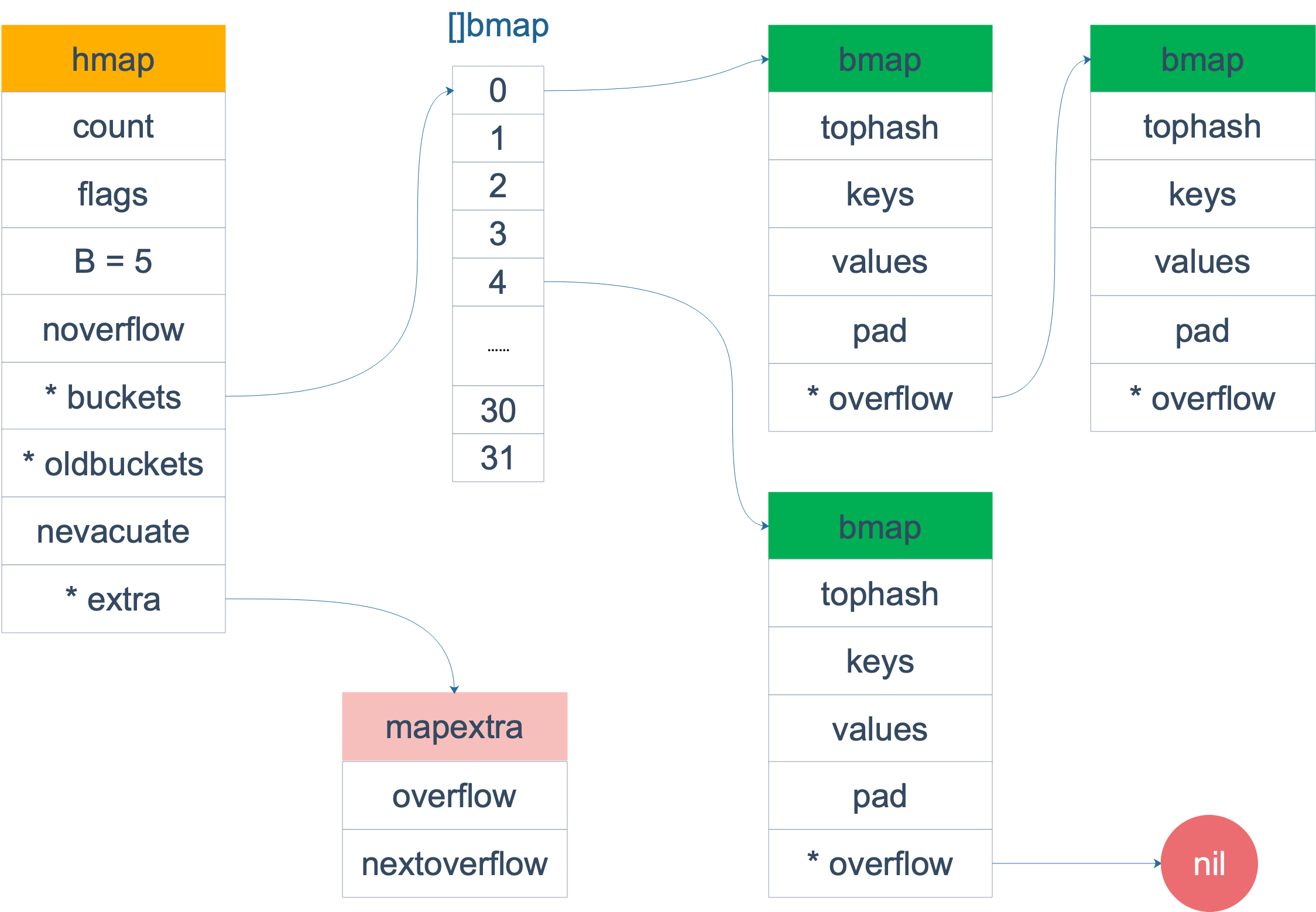``````type mapextra struct {
// overflow contains overflow buckets for hmap.buckets.
// overflow contains overflow buckets for hmap.oldbuckets.
overflow *[]*bmap

// nextOverflow 包含空闲的 overflow bucket，这是预分配的 bucket
nextOverflow *bmap
}
``````

bmap 是存放 k-v 的地方，我们把视角拉近，仔细看 bmap 的内部组成。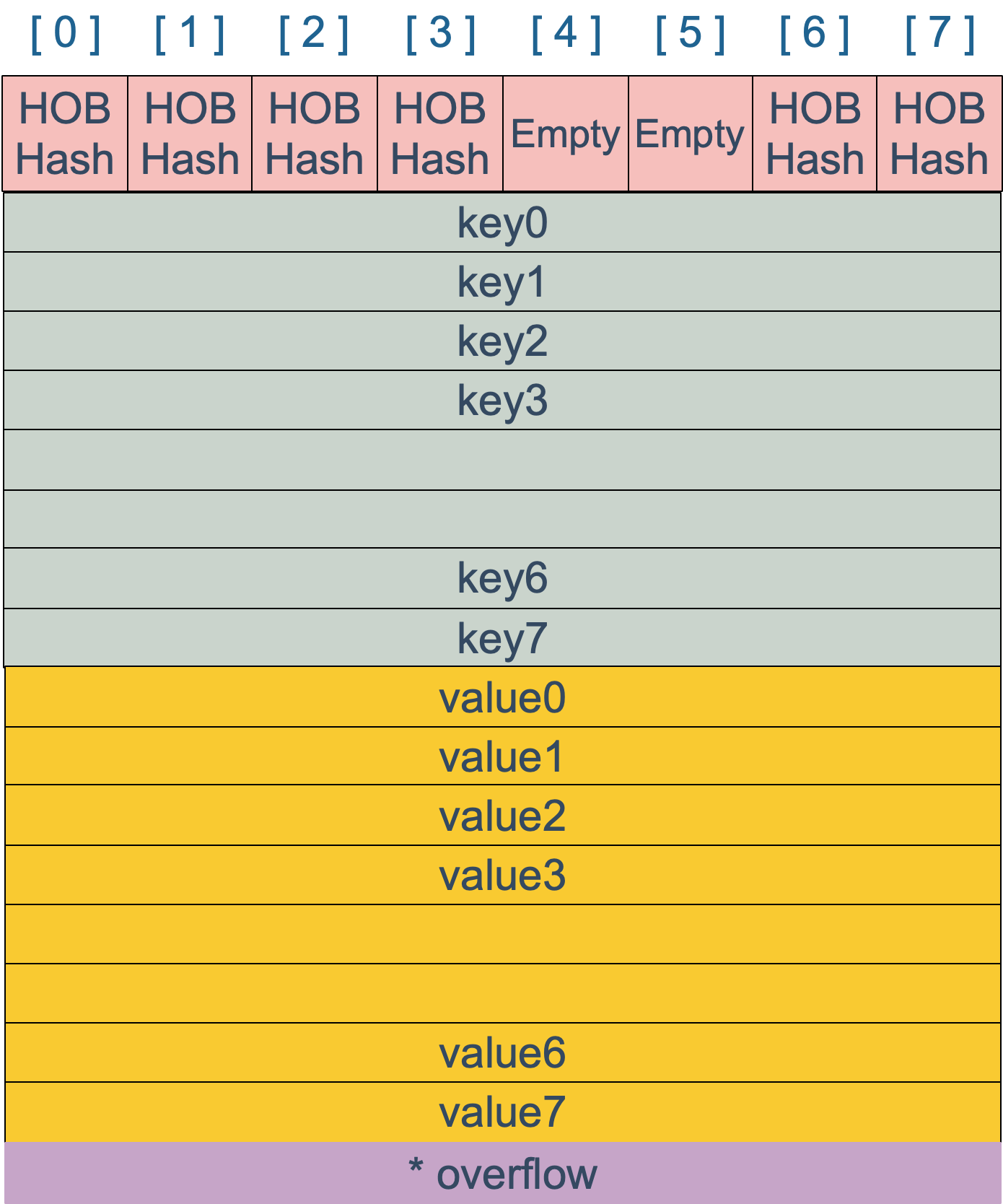``````map[int64]int8
``````

### 创建 map

``````ageMp := make(map[string]int)
// 指定 map 长度
ageMp := make(map[string]int, 8)

// ageMp 为 nil，不能向其添加元素，会直接panic
var ageMp map[string]int
``````

``````func makemap(t *maptype, hint int64, h *hmap, bucket unsafe.Pointer) *hmap {
// 省略各种条件检查...

// 找到一个 B，使得 map 的装载因子在正常范围内
B := uint8(0)
for ; overLoadFactor(hint, B); B++ {
}

// 初始化 hash table
// 如果 B 等于 0，那么 buckets 就会在赋值的时候再分配
// 如果长度比较大，分配内存会花费长一点
buckets := bucket
var extra *mapextra
if B != 0 {
var nextOverflow *bmap
buckets, nextOverflow = makeBucketArray(t, B)
if nextOverflow != nil {
extra = new(mapextra)
extra.nextOverflow = nextOverflow
}
}

// 初始化 hamp
if h == nil {
h = (*hmap)(newobject(t.hmap))
}
h.count = 0
h.B = B
h.extra = extra
h.flags = 0
h.hash0 = fastrand()
h.buckets = buckets
h.oldbuckets = nil
h.nevacuate = 0
h.noverflow = 0

return h
}
``````

``````func makeslice(et *_type, len, cap int) slice
``````

``````// runtime/slice.go
type slice struct {
array unsafe.Pointer // 元素指针
len   int // 长度
cap   int // 容量
}
``````

makemap 和 makeslice 的区别，带来一个不同点：当 map 和 slice 作为函数参数时，在函数参数内部对 map 的操作会影响 map 自身；而对 slice 却不会（之前讲 slice 的文章里有讲过）。

### 哈希函数

map 的一个关键点在于，哈希函数的选择。在程序启动时，会检测 cpu 是否支持 aes，如果支持，则使用 aes hash，否则使用 memhash。这是在函数 `alginit()` 中完成，位于路径：`src/runtime/alg.go` 下。

hash 函数，有加密型和非加密型。加密型的一般用于加密数据、数字摘要等，典型代表就是 md5、sha1、sha256、aes256 这种；非加密型的一般就是查找。在 map 的应用场景中，用的是查找。选择 hash 函数主要考察的是两点：性能、碰撞概率。

``````type _type struct {
size       uintptr
ptrdata    uintptr // size of memory prefix holding all pointers
hash       uint32
tflag      tflag
align      uint8
fieldalign uint8
kind       uint8
alg        *typeAlg
gcdata    *byte
str       nameOff
ptrToThis typeOff
}
``````

``````// src/runtime/alg.go
type typeAlg struct {
// (ptr to object, seed) -> hash
hash func(unsafe.Pointer, uintptr) uintptr
// (ptr to object A, ptr to object B) -> ==?
equal func(unsafe.Pointer, unsafe.Pointer) bool
}
``````

typeAlg 包含两个函数，hash 函数计算类型的哈希值，而 equal 函数则计算两个类型是否“哈希相等”。

``````func strhash(a unsafe.Pointer, h uintptr) uintptr {
x := (*stringStruct)(a)
return memhash(x.str, h, uintptr(x.len))
}

func strequal(p, q unsafe.Pointer) bool {
return *(*string)(p) == *(*string)(q)
}
``````

### key 定位过程

key 经过哈希计算后得到哈希值，共 64 个 bit 位（64位机，32位机就不讨论了，现在主流都是64位机），计算它到底要落在哪个桶时，只会用到最后 B 个 bit 位。还记得前面提到过的 B 吗？如果 B = 5，那么桶的数量，也就是 buckets 数组的长度是 2^5 = 32。

`````` 10010111 | 000011110110110010001111001010100010010110010101010 │ 01010
``````

buckets 编号就是桶编号，当两个不同的 key 落在同一个桶中，也就是发生了哈希冲突。冲突的解决手段是用链表法：在 bucket 中，从前往后找到第一个空位。这样，在查找某个 key 时，先找到对应的桶，再去遍历 bucket 中的 key。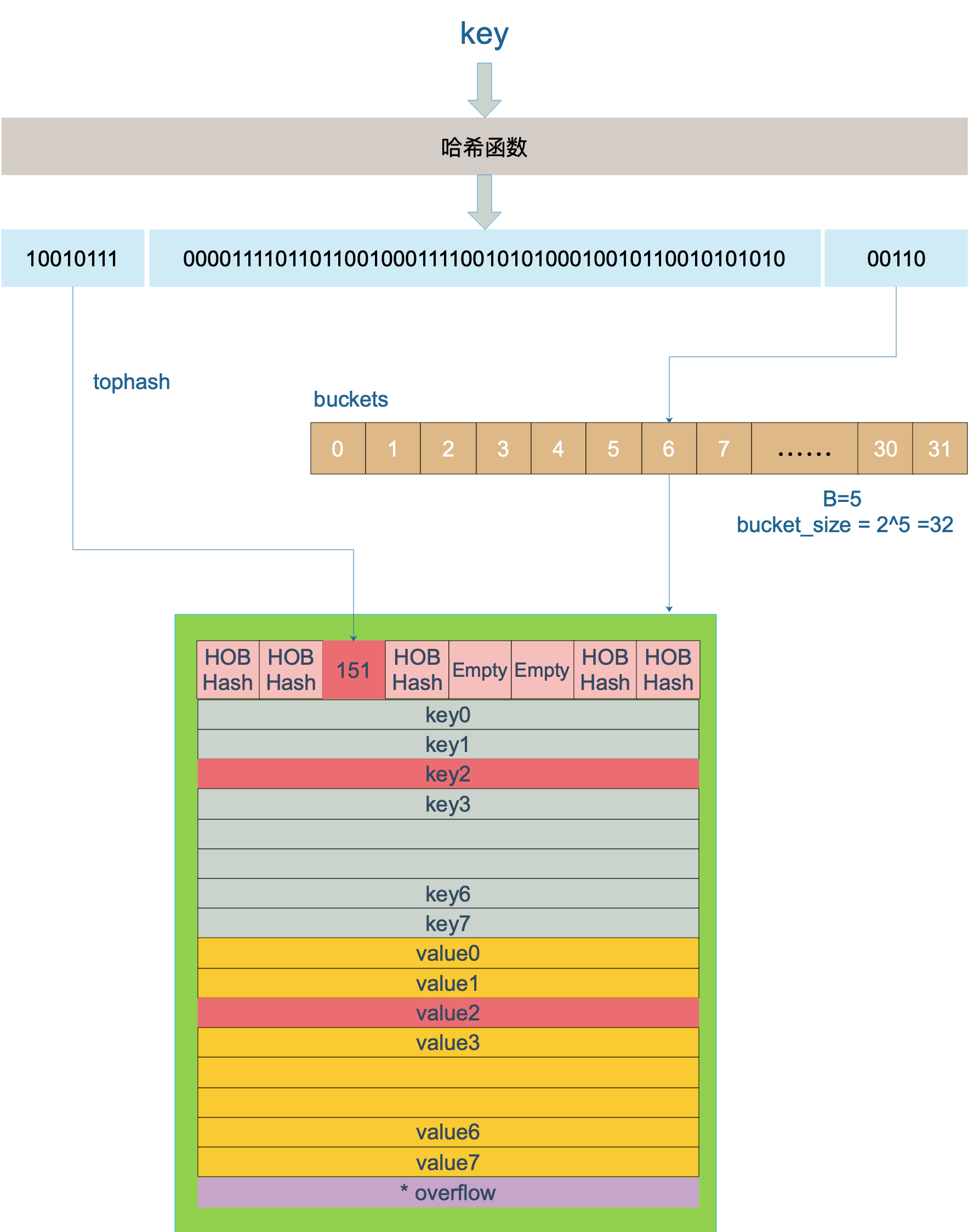``````func mapaccess1(t *maptype, h *hmap, key unsafe.Pointer) unsafe.Pointer {
// ……

// 如果 h 什么都没有，返回零值
if h == nil || h.count == 0 {
return unsafe.Pointer(&zeroVal)
}

// 写和读冲突
if h.flags&hashWriting != 0 {
throw("concurrent map read and map write")
}

// 不同类型 key 使用的 hash 算法在编译期确定
alg := t.key.alg

// 计算哈希值，并且加入 hash0 引入随机性
hash := alg.hash(key, uintptr(h.hash0))

// 比如 B=5，那 m 就是31，二进制是全 1
// 求 bucket num 时，将 hash 与 m 相与，
// 达到 bucket num 由 hash 的低 8 位决定的效果
m := uintptr(1)<<h.B - 1

// b 就是 bucket 的地址
b := (*bmap)(add(h.buckets, (hash&m)*uintptr(t.bucketsize)))

// oldbuckets 不为 nil，说明发生了扩容
if c := h.oldbuckets; c != nil {
// 如果不是同 size 扩容（看后面扩容的内容）
// 对应条件 1 的解决方案
if !h.sameSizeGrow() {
// 新 bucket 数量是老的 2 倍
m >>= 1
}

// 求出 key 在老的 map 中的 bucket 位置
oldb := (*bmap)(add(c, (hash&m)*uintptr(t.bucketsize)))

// 如果 oldb 没有搬迁到新的 bucket
// 那就在老的 bucket 中寻找
if !evacuated(oldb) {
b = oldb
}
}

// 计算出高 8 位的 hash
// 相当于右移 56 位，只取高8位
top := uint8(hash >> (sys.PtrSize*8 - 8))

// 增加一个 minTopHash
if top < minTopHash {
top += minTopHash
}
for {
// 遍历 8 个 bucket
for i := uintptr(0); i < bucketCnt; i++ {
// tophash 不匹配，继续
if b.tophash[i] != top {
continue
}
// tophash 匹配，定位到 key 的位置
k := add(unsafe.Pointer(b), dataOffset+i*uintptr(t.keysize))
// key 是指针
if t.indirectkey {
// 解引用
k = *((*unsafe.Pointer)(k))
}
// 如果 key 相等
if alg.equal(key, k) {
// 定位到 value 的位置
v := add(unsafe.Pointer(b), dataOffset+bucketCnt*uintptr(t.keysize)+i*uintptr(t.valuesize))
// value 解引用
if t.indirectvalue {
v = *((*unsafe.Pointer)(v))
}
return v
}
}

// bucket 找完（还没找到），继续到 overflow bucket 里找
b = b.overflow(t)
// overflow bucket 也找完了，说明没有目标 key
// 返回零值
if b == nil {
return unsafe.Pointer(&zeroVal)
}
}
}
``````

``````// key 定位公式
k := add(unsafe.Pointer(b), dataOffset+i*uintptr(t.keysize))

// value 定位公式
v := add(unsafe.Pointer(b), dataOffset+bucketCnt*uintptr(t.keysize)+i*uintptr(t.valuesize))
``````

b 是 bmap 的地址，这里 bmap 还是源码里定义的结构体，只包含一个 tophash 数组，经编译器扩充之后的结构体才包含 key，value，overflow 这些字段。dataOffset 是 key 相对于 bmap 起始地址的偏移：

``````b 是 bmap 的地址，这里 bmap 还是源码里定义的结构体，只包含一个 tophash 数组，经编译器扩充之后的结构体才包含 key，value，overflow 这些字段。dataOffset 是 key 相对于 bmap 起始地址的偏移：
``````

``````b = b.overflow(t)
``````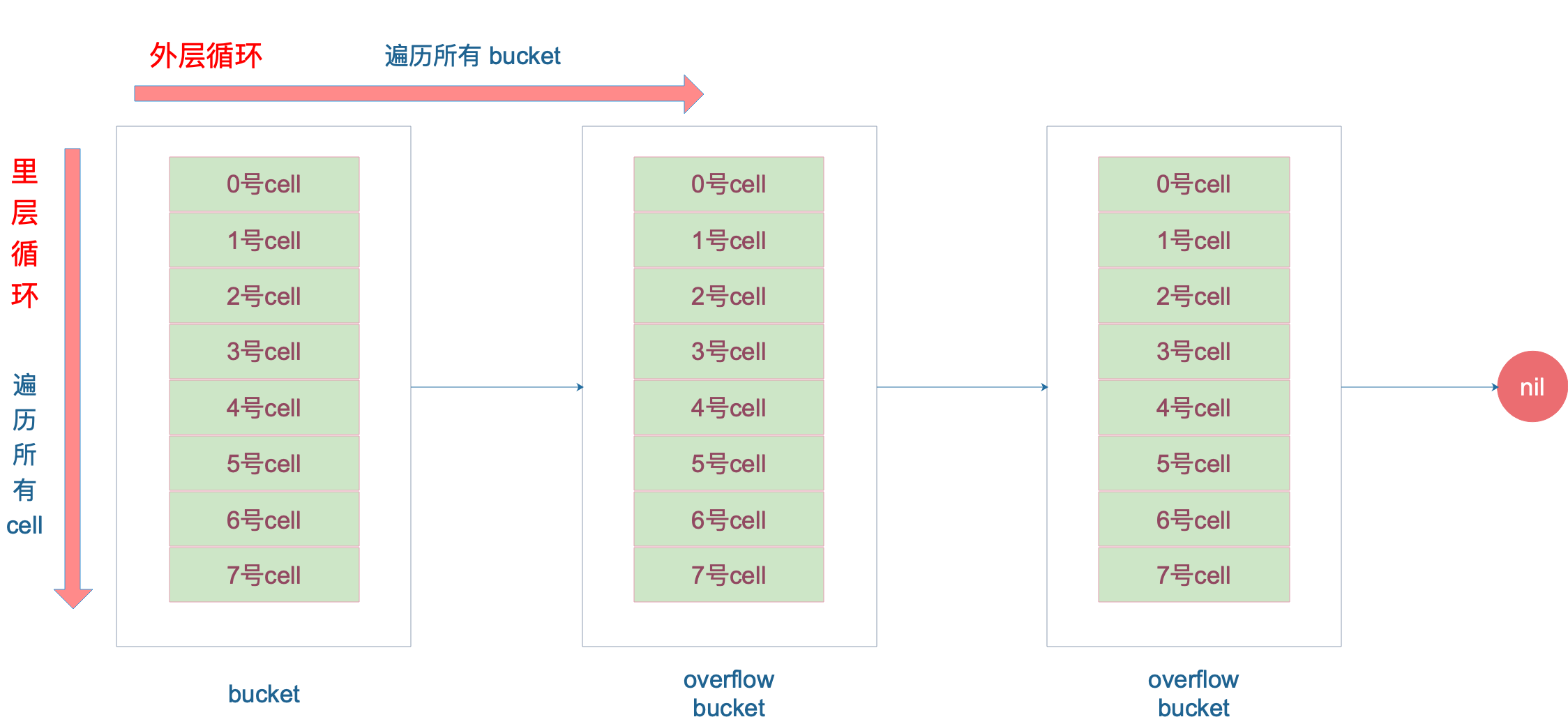``````// 空的 cell，也是初始时 bucket 的状态
empty          = 0
// 空的 cell，表示 cell 已经被迁移到新的 bucket
evacuatedEmpty = 1
// key,value 已经搬迁完毕，但是 key 都在新 bucket 前半部分，
// 后面扩容部分会再讲到。
evacuatedX     = 2
// 同上，key 在后半部分
evacuatedY     = 3
// tophash 的最小正常值
minTopHash     = 4
``````

``````func evacuated(b *bmap) bool {
h := b.tophash
return h > empty && h < minTopHash
}
``````

### map 的两种 get 操作

Go 语言中读取 map 有两种语法：带 comma 和 不带 comma。当要查询的 key 不在 map 里，带 comma 的用法会返回一个 bool 型变量提示 key 是否在 map 中；而不带 comma 的语句则会返回一个 value 类型的零值。如果 value 是 int 型就会返回 0，如果 value 是 string 类型，就会返回空字符串。

``````package main

import "fmt"

func main() {
ageMap := make(map[string]int)
ageMap["qcrao"] = 18

// 不带 comma 用法
age1 := ageMap["stefno"]
fmt.Println(age1)

// 带 comma 用法
age2, ok := ageMap["stefno"]
fmt.Println(age2, ok)
}
``````

``````0
0 false
``````

``````// src/runtime/hashmap.go
func mapaccess1(t *maptype, h *hmap, key unsafe.Pointer) unsafe.Pointer
func mapaccess2(t *maptype, h *hmap, key unsafe.Pointer) (unsafe.Pointer, bool)
``````

``````key 类型    查找
uint32    mapaccess1_fast32(t maptype, h hmap, key uint32) unsafe.Pointer
uint32    mapaccess2_fast32(t maptype, h hmap, key uint32) (unsafe.Pointer, bool)
uint64    mapaccess1_fast64(t maptype, h hmap, key uint64) unsafe.Pointer
uint64    mapaccess2_fast64(t maptype, h hmap, key uint64) (unsafe.Pointer, bool)
string    mapaccess1_faststr(t maptype, h hmap, ky string) unsafe.Pointer
string    mapaccess2_faststr(t maptype, h hmap, ky string) (unsafe.Pointer, bool)
``````

### 如何进行扩容

Go 语言采用一个 bucket 里装载 8 个 key，定位到某个 bucket 后，还需要再定位到具体的 key，这实际上又用了时间换空间。

``````loadFactor := count / (2^B)
``````

count 就是 map 的元素个数，2^B 表示 bucket 数量。

• 装载因子超过阈值，源码里定义的阈值是 6.5。
• overflow 的 bucket 数量过多：当 B 小于 15，也就是 bucket 总数 2^B 小于 2^15 时，如果 overflow 的 bucket 数量超过 2^B；当 B >= 15，也就是 bucket 总数 2^B 大于等于 2^15，如果 overflow 的 bucket 数量超过 2^15。

``````// src/runtime/hashmap.go/mapassign

// 触发扩容时机
if !h.growing() && (overLoadFactor(int64(h.count), h.B) || tooManyOverflowBuckets(h.noverflow, h.B)) {
hashGrow(t, h)
}

// 装载因子超过 6.5
func overLoadFactor(count int64, B uint8) bool {
return count >= bucketCnt && float32(count) >= loadFactor*float32((uint64(1)<<B))
}

// overflow buckets 太多
func tooManyOverflowBuckets(noverflow uint16, B uint8) bool {
if B < 16 {
return noverflow >= uint16(1)<<B
}
return noverflow >= 1<<15
}
``````

``````func hashGrow(t *maptype, h *hmap) {
// B+1 相当于是原来 2 倍的空间
bigger := uint8(1)

// 对应条件 2
if !overLoadFactor(int64(h.count), h.B) {
// 进行等量的内存扩容，所以 B 不变
bigger = 0
h.flags |= sameSizeGrow
}
// 将老 buckets 挂到 buckets 上
oldbuckets := h.buckets
// 申请新的 buckets 空间
newbuckets, nextOverflow := makeBucketArray(t, h.B+bigger)

flags := h.flags &^ (iterator | oldIterator)
if h.flags&iterator != 0 {
flags |= oldIterator
}
// 提交 grow 的动作
h.B += bigger
h.flags = flags
h.oldbuckets = oldbuckets
h.buckets = newbuckets
// 搬迁进度为 0
h.nevacuate = 0
// overflow buckets 数为 0
h.noverflow = 0

// ……
}
``````

``````flags := h.flags &^ (iterator | oldIterator)
if h.flags&iterator != 0 {
flags |= oldIterator
}
``````

``````x = 01010011
y = 01010100
z = x &^ y = 00000011
``````

``````/ 可能有迭代器使用 buckets
iterator     = 1
// 可能有迭代器使用 oldbuckets
oldIterator  = 2
// 有协程正在向 map 中写入 key
hashWriting  = 4
// 等量扩容（对应条件 2）
sameSizeGrow = 8
``````

``````func growWork(t *maptype, h *hmap, bucket uintptr) {
// 确认搬迁老的 bucket 对应正在使用的 bucket

// 再搬迁一个 bucket，以加快搬迁进程
if h.growing() {
evacuate(t, h, h.nevacuate)
}
}
``````

h.growing() 函数非常简单：

``````func (h *hmap) growing() bool {
return h.oldbuckets != nil
}
``````

`bucket&h.oldbucketmask()` 这行代码，如源码注释里说的，是为了确认搬迁的 bucket 是我们正在使用的 bucket。`oldbucketmask()` 函数返回扩容前的 map 的 bucketmask。

``````func evacuate(t *maptype, h *hmap, oldbucket uintptr) {
// 定位老的 bucket 地址
b := (*bmap)(add(h.oldbuckets, oldbucket*uintptr(t.bucketsize)))
// 结果是 2^B，如 B = 5，结果为32
newbit := h.noldbuckets()
// key 的哈希函数
alg := t.key.alg
// 如果 b 没有被搬迁过
if !evacuated(b) {
var (
// 表示bucket 移动的目标地址
x, y   *bmap
// 指向 x,y 中的 key/val
xi, yi int
// 指向 x，y 中的 key
xk, yk unsafe.Pointer
// 指向 x，y 中的 value
xv, yv unsafe.Pointer
)
// 默认是等 size 扩容，前后 bucket 序号不变
// 使用 x 来进行搬迁
x = (*bmap)(add(h.buckets, oldbucket*uintptr(t.bucketsize)))
xi = 0
xk = add(unsafe.Pointer(x), dataOffset)
xv = add(xk, bucketCnt*uintptr(t.keysize))、

// 如果不是等 size 扩容，前后 bucket 序号有变
// 使用 y 来进行搬迁
if !h.sameSizeGrow() {
// y 代表的 bucket 序号增加了 2^B
y = (*bmap)(add(h.buckets, (oldbucket+newbit)*uintptr(t.bucketsize)))
yi = 0
yk = add(unsafe.Pointer(y), dataOffset)
yv = add(yk, bucketCnt*uintptr(t.keysize))
}

// 遍历所有的 bucket，包括 overflow buckets
// b 是老的 bucket 地址
for ; b != nil; b = b.overflow(t) {
k := add(unsafe.Pointer(b), dataOffset)
v := add(k, bucketCnt*uintptr(t.keysize))

// 遍历 bucket 中的所有 cell
for i := 0; i < bucketCnt; i, k, v = i+1, add(k, uintptr(t.keysize)), add(v, uintptr(t.valuesize)) {
// 当前 cell 的 top hash 值
top := b.tophash[i]
// 如果 cell 为空，即没有 key
if top == empty {
// 那就标志它被"搬迁"过
b.tophash[i] = evacuatedEmpty
// 继续下个 cell
continue
}
// 正常不会出现这种情况
// 未被搬迁的 cell 只可能是 empty 或是
// 正常的 top hash（大于 minTopHash）
if top < minTopHash {
}

k2 := k
// 如果 key 是指针，则解引用
if t.indirectkey {
k2 = *((*unsafe.Pointer)(k2))
}

// 默认使用 X，等量扩容
useX := true
// 如果不是等量扩容
if !h.sameSizeGrow() {
// 计算 hash 值，和 key 第一次写入时一样
hash := alg.hash(k2, uintptr(h.hash0))

// 如果有协程正在遍历 map
if h.flags&iterator != 0 {
// 如果出现 相同的 key 值，算出来的 hash 值不同
if !t.reflexivekey && !alg.equal(k2, k2) {
// 只有在 float 变量的 NaN() 情况下会出现
if top&1 != 0 {
// 第 B 位置 1
hash |= newbit
} else {
// 第 B 位置 0
hash &^= newbit
}
// 取高 8 位作为 top hash 值
top = uint8(hash >> (sys.PtrSize*8 - 8))
if top < minTopHash {
top += minTopHash
}
}
}

// 取决于新哈希值的 oldB+1 位是 0 还是 1
// 详细看后面的文章
useX = hash&newbit == 0
}

// 如果 key 搬到 X 部分
if useX {
// 标志老的 cell 的 top hash 值，表示搬移到 X 部分
b.tophash[i] = evacuatedX
// 如果 xi 等于 8，说明要溢出了
if xi == bucketCnt {
// 新建一个 bucket
newx := h.newoverflow(t, x)
x = newx
// xi 从 0 开始计数
xi = 0
// xk 表示 key 要移动到的位置
xk = add(unsafe.Pointer(x), dataOffset)
// xv 表示 value 要移动到的位置
xv = add(xk, bucketCnt*uintptr(t.keysize))
}
// 设置 top hash 值
x.tophash[xi] = top
// key 是指针
if t.indirectkey {
// 将原 key（是指针）复制到新位置
*(*unsafe.Pointer)(xk) = k2 // copy pointer
} else {
// 将原 key（是值）复制到新位置
typedmemmove(t.key, xk, k) // copy value
}
// value 是指针，操作同 key
if t.indirectvalue {
*(*unsafe.Pointer)(xv) = *(*unsafe.Pointer)(v)
} else {
typedmemmove(t.elem, xv, v)
}

// 定位到下一个 cell
xi++
xk = add(xk, uintptr(t.keysize))
xv = add(xv, uintptr(t.valuesize))
} else { // key 搬到 Y 部分，操作同 X 部分
// ……
// 省略了这部分，操作和 X 部分相同
}
}
}
// 如果没有协程在使用老的 buckets，就把老 buckets 清除掉，帮助gc
if h.flags&oldIterator == 0 {
b = (*bmap)(add(h.oldbuckets, oldbucket*uintptr(t.bucketsize)))
// 只清除bucket 的 key,value 部分，保留 top hash 部分，指示搬迁状态
if t.bucket.kind&kindNoPointers == 0 {
} else {
}
}
}

// 更新搬迁进度
// 如果此次搬迁的 bucket 等于当前进度
if oldbucket == h.nevacuate {
// 进度加 1
h.nevacuate = oldbucket + 1
// Experiments suggest that 1024 is overkill by at least an order of magnitude.
// Put it in there as a safeguard anyway, to ensure O(1) behavior.
// 尝试往后看 1024 个 bucket
stop := h.nevacuate + 1024
if stop > newbit {
stop = newbit
}
// 寻找没有搬迁的 bucket
for h.nevacuate != stop && bucketEvacuated(t, h, h.nevacuate) {
h.nevacuate++
}

// 现在 h.nevacuate 之前的 bucket 都被搬迁完毕

// 所有的 buckets 搬迁完毕
if h.nevacuate == newbit {
// 清除老的 buckets
h.oldbuckets = nil
// 清除老的 overflow bucket
// 回忆一下： 表示当前 overflow bucket
//  表示 old overflow bucket
if h.extra != nil {
h.extra.overflow = nil
}
// 清除正在扩容的标志位
h.flags &^= sameSizeGrow
}
}
}
``````

evacuate 函数的代码注释非常清晰，对着代码和注释是很容易看懂整个的搬迁过程的，耐心点。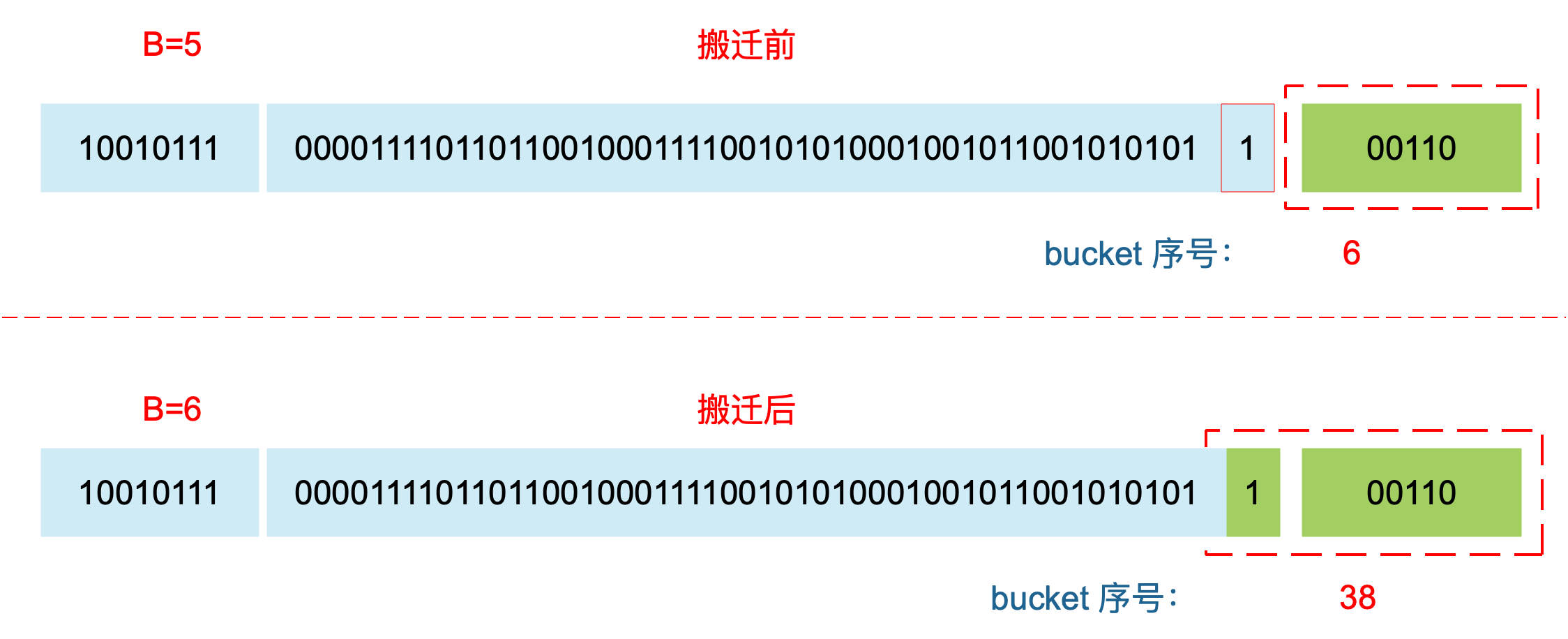map 在扩容后，会发生 key 的搬迁，原来落在同一个 bucket 中的 key，搬迁后，有些 key 就要远走高飞了（bucket 序号加上了 2^B）。而遍历的过程，就是按顺序遍历 bucket，同时按顺序遍历 bucket 中的 key。搬迁后，key 的位置发生了重大的变化，有些 key 飞上高枝，有些 key 则原地不动。这样，遍历 map 的结果就不可能按原来的顺序了。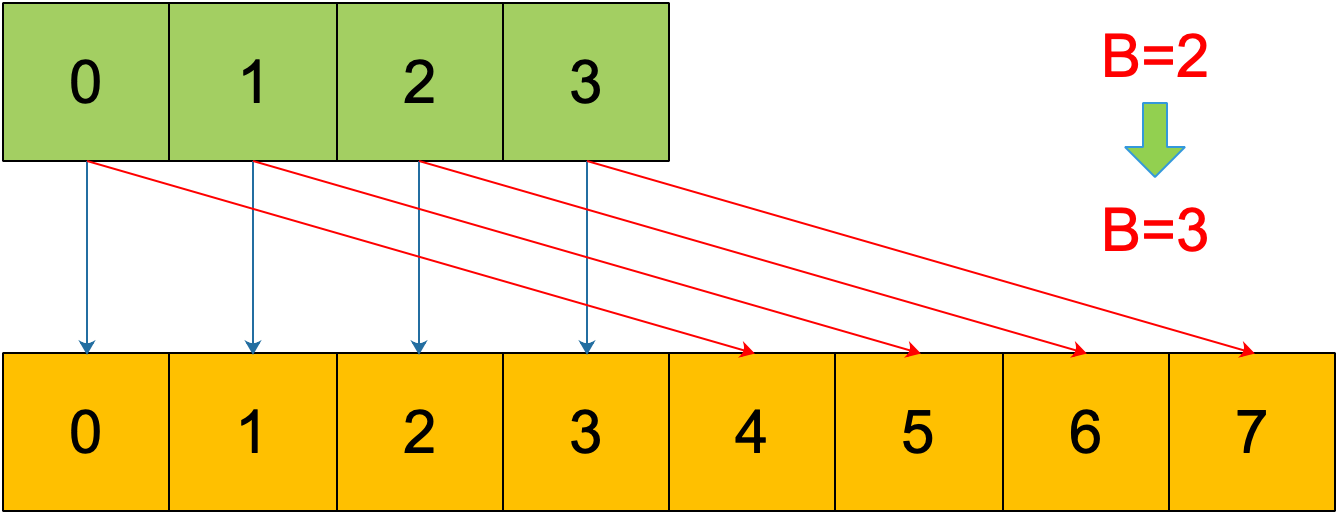evacuate 函数每次只完成一个 bucket 的搬迁工作，因此要遍历完此 bucket 的所有的 cell，将有值的 cell copy 到新的地方。bucket 还会链接 overflow bucket，它们同样需要搬迁。因此会有 2 层循环，外层遍历 bucket 和 overflow bucket，内层遍历 bucket 的所有 cell。这样的循环在 map 的源码里到处都是，要理解透了。

``````if top&1 != 0 {
// top hash 最低位为 1
// 新算出来的 hash 值的 B 位置 1
hash |= newbit
} else {
// 新算出来的 hash 值的 B 位置 0
hash &^= newbit
}

// hash 值的 B 位为 0，则搬迁到 x part
// 当 B = 5时，newbit = 32，二进制低 6 位为 10 0000
useX = hash&newbit == 0
``````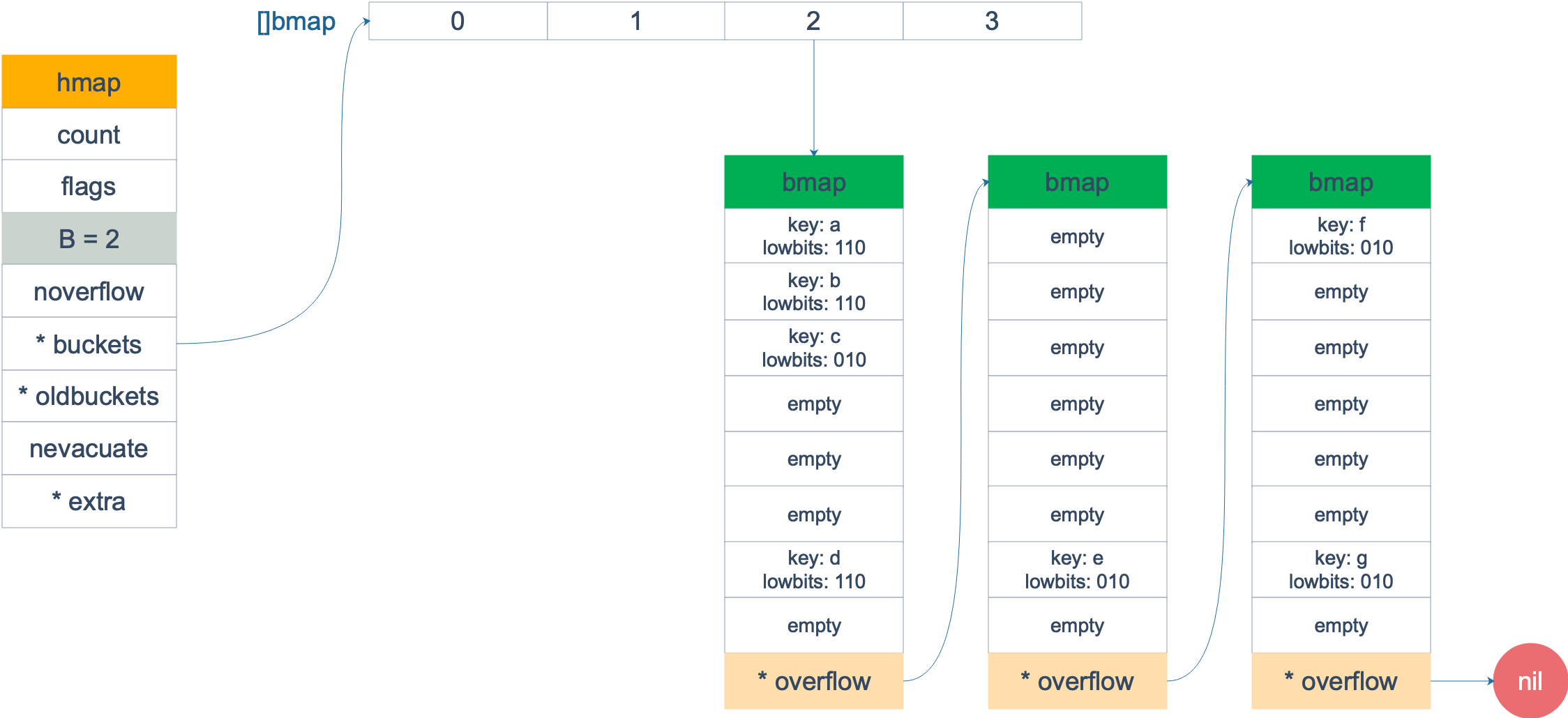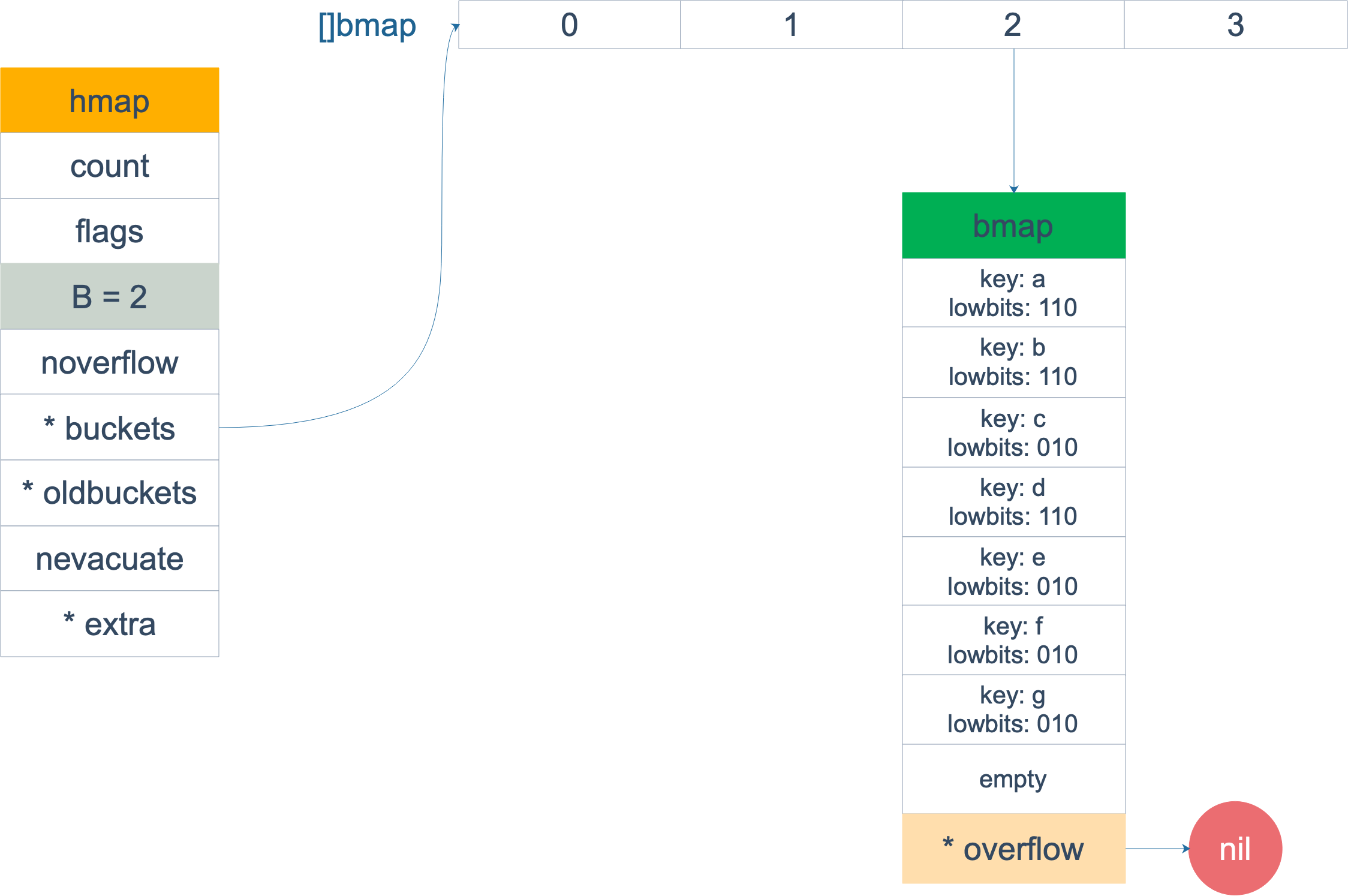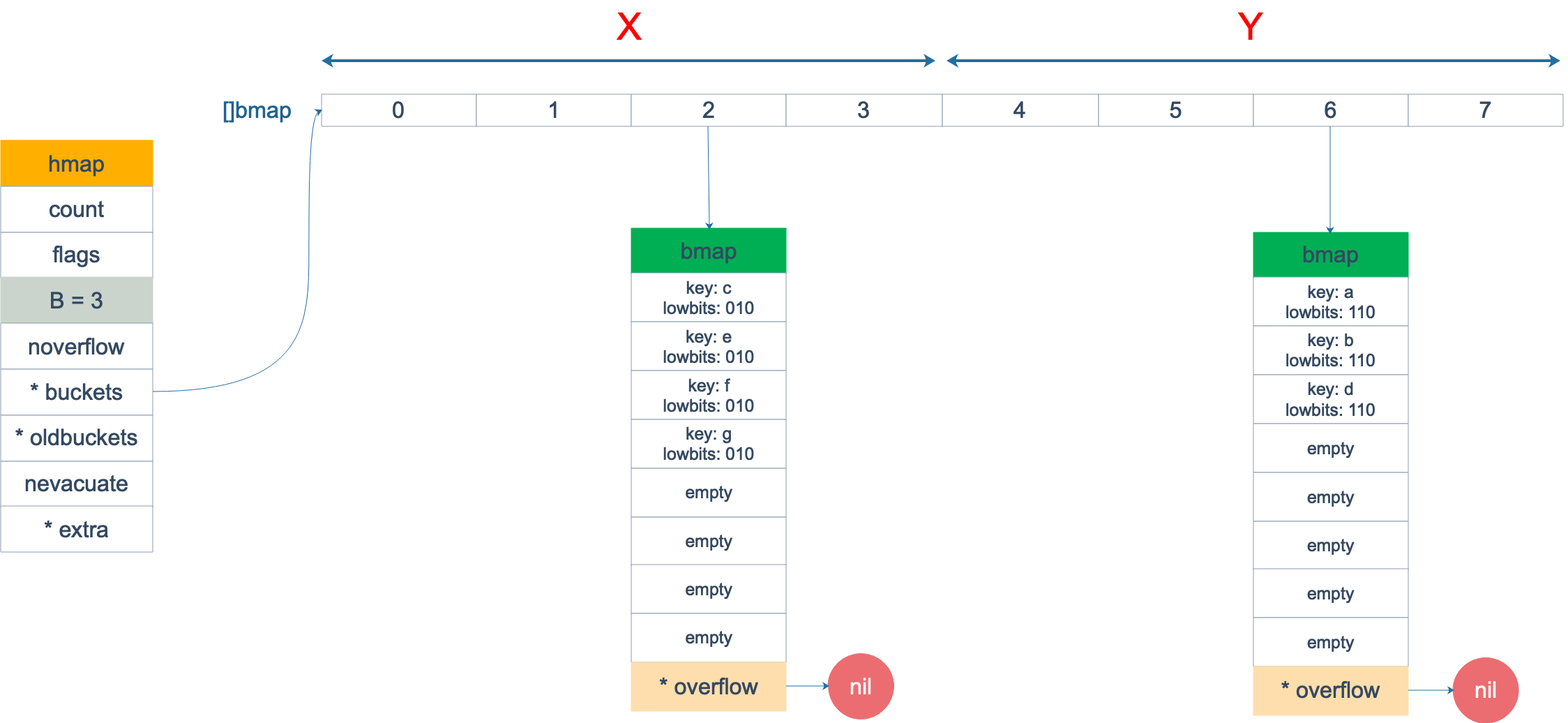### map 的遍历

``````package main

import "fmt"

func main() {
ageMp := make(map[string]int)
ageMp["qcrao"] = 18

for name, age := range ageMp {
fmt.Println(name, age)
}
}
``````

``````go tool compile -S main.go
``````

``````// ......
0x0124 00292 (test16.go:9)      CALL    runtime.mapiterinit(SB)

// ......
0x01fb 00507 (test16.go:9)      CALL    runtime.mapiternext(SB)
0x0200 00512 (test16.go:9)      MOVQ    ""..autotmp_4+160(SP), AX
0x0208 00520 (test16.go:9)      TESTQ   AX, AX
0x020b 00523 (test16.go:9)      JNE     302

// ......
``````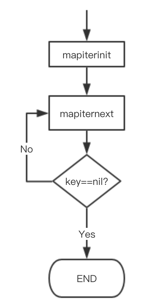``````type hiter struct {
// key 指针
key         unsafe.Pointer
// value 指针
value       unsafe.Pointer
// map 类型，包含如 key size 大小等
t           *maptype
h           *hmap
// 初始化时指向的 bucket
buckets     unsafe.Pointer
// 当前遍历到的 bmap
bptr        *bmap
overflow    *[]*bmap
// 起始遍历的 bucet 编号
startBucket uintptr
// 遍历开始时 cell 的编号（每个 bucket 中有 8 个 cell）
offset      uint8
// 是否从头遍历了
wrapped     bool
// B 的大小
B           uint8
// 指示当前 cell 序号
i           uint8
// 指向当前的 bucket
bucket      uintptr
// 因为扩容，需要检查的 bucket
checkBucket uintptr
}
``````

`mapiterinit` 就是对 hiter 结构体里的字段进行初始化赋值操作。

``````// 生成随机数 r
r := uintptr(fastrand())
if h.B > 31-bucketCntBits {
r += uintptr(fastrand()) << 31
}

// 从哪个 bucket 开始遍历
it.startBucket = r & (uintptr(1)<<h.B - 1)
// 从 bucket 的哪个 cell 开始遍历
it.offset = uint8(r >> h.B & (bucketCnt - 1))
``````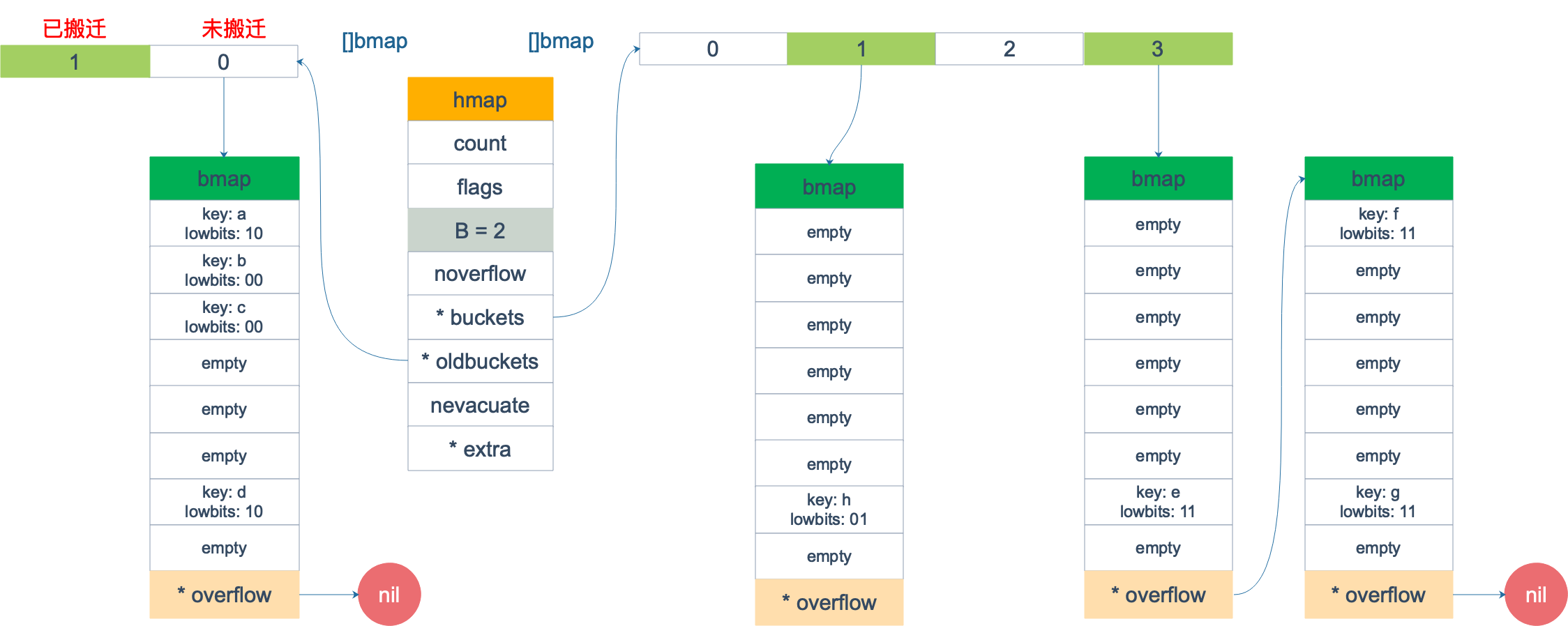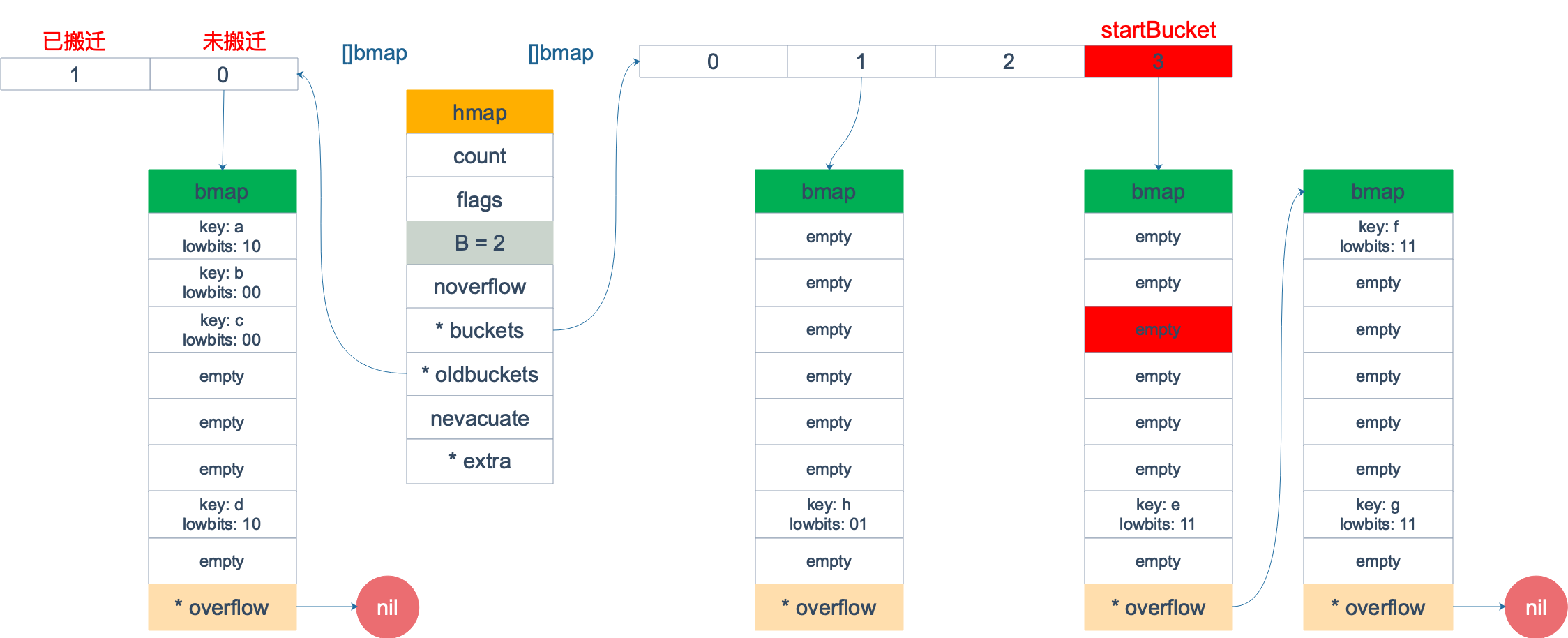``````func evacuated(b *bmap) bool {
h := b.tophash
return h > empty && h < minTopHash
}
``````

``````empty = 0
evacuatedEmpty = 1
evacuatedX = 2
evacuatedY = 3
minTopHash = 4
``````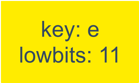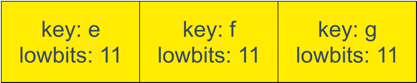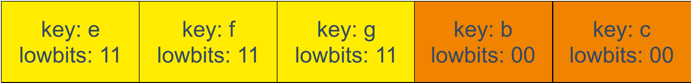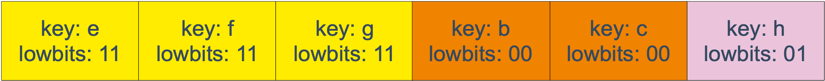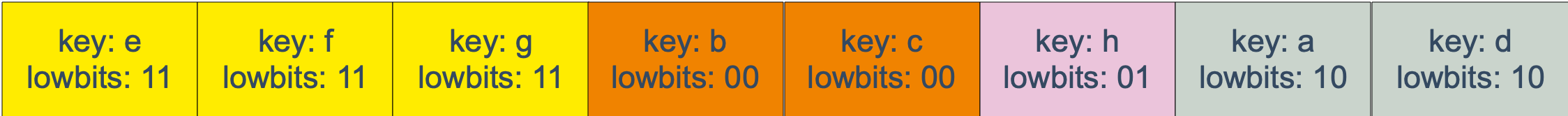map 遍历的核心在于理解 2 倍扩容时，老 bucket 会分裂到 2 个新 bucket 中去。而遍历操作，会按照新 bucket 的序号顺序进行，碰到老 bucket 未搬迁的情况时，要在老 bucket 中找到将来要搬迁到新 bucket 来的 key。

### map 的赋值

mapassign 有一个系列的函数，根据 key 类型的不同，编译器会将其优化为相应的“快速函数”。

``````key 类型    插入
uint32    mapassign_fast32(t maptype, h hmap, key uint32) unsafe.Pointer
uint64    mapassign_fast64(t maptype, h hmap, key uint64) unsafe.Pointer
string    mapassign_faststr(t maptype, h hmap, ky string) unsafe.Pointer
``````

``````func mapassign(t *maptype, h *hmap, key unsafe.Pointer) unsafe.Pointer
``````

### map的删除

``````func mapdelete(t *maptype, h *hmap, key unsafe.Pointer)
``````

``````key 类型    删除
uint32    mapdelete_fast32(t maptype, h hmap, key uint32)
uint64    mapdelete_fast64(t maptype, h hmap, key uint64)
string    mapdelete_faststr(t maptype, h hmap, ky string)
``````

``````// 对 key 清零
if t.indirectkey {
*(*unsafe.Pointer)(k) = nil
} else {
typedmemclr(t.key, k)
}

// 对 value 清零
if t.indirectvalue {
*(*unsafe.Pointer)(v) = nil
} else {
typedmemclr(t.elem, v)
}
``````

## map进阶

### key可以是float型吗？

``````func main() {
m := make(map[float64]int)
m[1.4] = 1
m[2.4] = 2
m[math.NaN()] = 3
m[math.NaN()] = 3

for k, v := range m {
fmt.Printf("[%v, %d] ", k, v)
}

fmt.Printf("\nk: %v, v: %d\n", math.NaN(), m[math.NaN()])
fmt.Printf("k: %v, v: %d\n", 2.400000000001, m[2.400000000001])
fmt.Printf("k: %v, v: %d\n", 2.4000000000000000000000001, m[2.4000000000000000000000001])

fmt.Println(math.NaN() == math.NaN())
}
``````

``````[2.4, 2] [NaN, 3] [NaN, 3] [1.4, 1]
k: NaN, v: 0
k: 2.400000000001, v: 0
k: 2.4, v: 2
false
``````

``````// Float64frombits returns the floating point number corresponding
// the IEEE 754 binary representation b.
func Float64frombits(b uint64) float64 { return *(*float64)(unsafe.Pointer(&b)) }
``````

``````0x00bd 00189 (test18.go:9)      LEAQ    "".statictmp_0(SB), DX
0x00c4 00196 (test18.go:9)      MOVQ    DX, 16(SP)
0x00c9 00201 (test18.go:9)      PCDATA  \$0, \$2
0x00c9 00201 (test18.go:9)      CALL    runtime.mapassign(SB)
``````

`"".statictmp_0(SB)` 变量是这样的：

``````"".statictmp_0 SRODATA size=8
0x0000 33 33 33 33 33 33 03 40
"".statictmp_1 SRODATA size=8
0x0000 ff 3b 33 33 33 33 03 40
"".statictmp_2 SRODATA size=8
0x0000 33 33 33 33 33 33 03 40
``````

``````package main

import (
"fmt"
"math"
)

func main() {
m := make(map[float64]int)
m[2.4] = 2

fmt.Println(math.Float64bits(2.4))
fmt.Println(math.Float64bits(2.400000000001))
fmt.Println(math.Float64bits(2.4000000000000000000000001))
}
``````
``````4612586738352864255
4612586738352862003
4612586738352862003
``````

``````0x4003333333333333
0x4003333333333BFF
0x4003333333333333
``````

``````// NaN returns an IEEE 754 ``not-a-number'' value.
func NaN() float64 { return Float64frombits(uvnan) }
``````

uvan 的定义为：

``````uvnan    = 0x7FF8000000000001
``````

NAN() 直接调用 `Float64frombits`，传入写死的 const 型变量 `0x7FF8000000000001`，得到 NAN 型值。既然，NAN 是从一个常量解析得来的，为什么插入 map 时，会被认为是不同的 key？

``````func f64hash(p unsafe.Pointer, h uintptr) uintptr {
f := *(*float64)(p)
switch {
case f == 0:
return c1 * (c0 ^ h) // +0, -0
case f != f:
return c1 * (c0 ^ h ^ uintptr(fastrand())) // any kind of NaN
default:
return memhash(p, h, 8)
}
}
``````

``````NAN != NAN
hash(NAN) != hash(NAN)
``````

## 参考资料

【english 如何实现随机取一个map的key，非常精彩】https://lukechampine.com/hackmap.html

【各种 map 相关操作的流程图】https://www.jianshu.com/p/aa0d4808cbb8

【曹大关于map的文章 不用解释】https://github.com/cch123/golang-notes/blob/master/map.md

【english 对比了 java, c++ 的 map 实现】https://dave.cheney.net/2018/05/29/how-the-go-runtime-implements-maps-efficiently-without-generics

【english 为什么 go map 对竞争敏感】https://dave.cheney.net/2015/12/07/are-go-maps-sensitive-to-data-races

【golang blog map】https://blog.golang.org/go-maps-in-action

【randommap 开源代码】https://github.com/lukechampine/randmap

【新发现的博客，很有深度】https://draveness.me/golang-hashmap

【map 遍历 源码简单阐述】https://gocn.vip/article/1704

【短文，同时遍历、删除key是可以的】https://cloud.tencent.com/developer/article/1065474

【面向信仰编程，golang range】https://draveness.me/golang-for-range

【Go官方博客关于 map】https://blog.golang.org/go-maps-in-action

【Go 语言可比较类型】https://golang.org/ref/spec#Comparison_operators

【哈希函数选择，C++/Java 对比】https://studygolang.com/articles/15839

【如何比较两个 map 相等】https://golangbot.com/maps/

【NAN hash】https://research.swtch.com/randhash

Reprint please specify: KYLE LIU 【转】深度解密Go语言之 map

Previous【转】深度解密Go语言之unsafe

2019-06-03
NextGolnag在现实世界的并发错误【翻译】

2019-05-19
TOC